RRB Group D Mock Test - 29

# RRB Group D Mock Test - 29

Test Description

## 100 Questions MCQ Test RRB (Group D) - Mock Tests & Previous Year Papers | RRB Group D Mock Test - 29

RRB Group D Mock Test - 29 for Railways 2022 is part of RRB (Group D) - Mock Tests & Previous Year Papers preparation. The RRB Group D Mock Test - 29 questions and answers have been prepared according to the Railways exam syllabus.The RRB Group D Mock Test - 29 MCQs are made for Railways 2022 Exam. Find important definitions, questions, notes, meanings, examples, exercises, MCQs and online tests for RRB Group D Mock Test - 29 below.
Solutions of RRB Group D Mock Test - 29 questions in English are available as part of our RRB (Group D) - Mock Tests & Previous Year Papers for Railways & RRB Group D Mock Test - 29 solutions in Hindi for RRB (Group D) - Mock Tests & Previous Year Papers course. Download more important topics, notes, lectures and mock test series for Railways Exam by signing up for free. Attempt RRB Group D Mock Test - 29 | 100 questions in 90 minutes | Mock test for Railways preparation | Free important questions MCQ to study RRB (Group D) - Mock Tests & Previous Year Papers for Railways Exam | Download free PDF with solutions
 1 Crore+ students have signed up on EduRev. Have you?
RRB Group D Mock Test - 29 - Question 1

### International Bird Festival recently concluded in February 2018 in which of the following National Parks?

RRB Group D Mock Test - 29 - Question 2

### Who is Michael Phelps?

RRB Group D Mock Test - 29 - Question 3

### Which of the following primates are very poor tree climbers?

RRB Group D Mock Test - 29 - Question 4

Which is the Indian state which produces the most amount of rice?

RRB Group D Mock Test - 29 - Question 5

Which of the following animals found in India is on the list of Endangered Animals Status?

RRB Group D Mock Test - 29 - Question 6

Which of the following countries is a member of the OECD - Organisation for Economic Cooperation and Development?

RRB Group D Mock Test - 29 - Question 7

”City of Joy” is the nickname of a city in which Indian state?

RRB Group D Mock Test - 29 - Question 8

Oviparous organisms are those that ….

RRB Group D Mock Test - 29 - Question 9

”Avian Dinosaurs” are commonly referred to as ….

RRB Group D Mock Test - 29 - Question 10

Dum Dum International Airport is located in which city?

RRB Group D Mock Test - 29 - Question 11

In which year did Sikkim join the Indian Republic?

RRB Group D Mock Test - 29 - Question 12

Which of the following is NOT one of the great observatories of NASA?

RRB Group D Mock Test - 29 - Question 13

How many Indians have been to space so far?

RRB Group D Mock Test - 29 - Question 14

Which of the following cities is the farthest away from the Tropic of Cancer?

RRB Group D Mock Test - 29 - Question 15

Ghoomar is the classical dance of which state?

RRB Group D Mock Test - 29 - Question 16

In election commission, how many members are there currently?

RRB Group D Mock Test - 29 - Question 17

The type of force that acts between two dissimilar objects is called …

RRB Group D Mock Test - 29 - Question 18

Which is the lightest metal?Report Question

RRB Group D Mock Test - 29 - Question 19

Who has recently taken over as the new Chief of Staff Western Naval Command in January 2018?

RRB Group D Mock Test - 29 - Question 20

Ashes trophy is played between countries?

RRB Group D Mock Test - 29 - Question 21

Which of the following diseases can be prevented by vaccines?

RRB Group D Mock Test - 29 - Question 22

Which of the following cities in India produces the highest quantity of leather?

RRB Group D Mock Test - 29 - Question 23

Some reptiles like frogs and crocodiles bask in the sun to maintain their body temperature. This is the reason why they are called …

RRB Group D Mock Test - 29 - Question 24

Which of the following countries is not a member of the NSG -Nuclear Suppliers Group?

RRB Group D Mock Test - 29 - Question 25

India’s longest railway platform is the …

RRB Group D Mock Test - 29 - Question 26

The phenomenon by which fine, fertile soil gets swept away by flowing water leaving the land unsuitable for agriculture is called ...

RRB Group D Mock Test - 29 - Question 27

A close association between two or more organisms belonging to different species that helps in the survival of each of them is called …

RRB Group D Mock Test - 29 - Question 28

Where is the headquarters of “The Hindu”?

RRB Group D Mock Test - 29 - Question 29

Which of the following cities is the southernmost in India?

RRB Group D Mock Test - 29 - Question 30

Who is the third astronaut who traveled to the Moon along with Neil Armstrong and Buzz Aldrin?

RRB Group D Mock Test - 29 - Question 31

How fast do raindrops fall to the ground?

RRB Group D Mock Test - 29 - Question 32

Which of the following city called as “City of Seven Islands”?

RRB Group D Mock Test - 29 - Question 33

Who among the following is famous Indian classical dancer and theosophist?

RRB Group D Mock Test - 29 - Question 34

Which article of India constitution provides for election commission?

RRB Group D Mock Test - 29 - Question 35

We all know that Lotus Temple is the famous temple located in New Delhi it is open for which religion?

RRB Group D Mock Test - 29 - Question 36

Phobos is a moon of which of the following planet?

RRB Group D Mock Test - 29 - Question 37

Which of the following is the correct Abbreviation of PACS?

RRB Group D Mock Test - 29 - Question 38

Which of the following is a metal which is in liquid state at room temperature?

RRB Group D Mock Test - 29 - Question 39

What is the chemical present in plants that is essential for photosynthesis to happen?

RRB Group D Mock Test - 29 - Question 40

ich of the following is the correct Abbreviation of NSSO?

RRB Group D Mock Test - 29 - Question 41

Who is the present Director General of International Atomic Energy Agency- IAEA?

RRB Group D Mock Test - 29 - Question 42

Which of the following certainly don’t have tails?

RRB Group D Mock Test - 29 - Question 43

The idea of “Lokpal” bill is taken from?

RRB Group D Mock Test - 29 - Question 44

Who was the last Mughal emperor?

RRB Group D Mock Test - 29 - Question 45

The brain and the spinal cord are the major organs belonging to the … system.

RRB Group D Mock Test - 29 - Question 46

Which one of the following petroleum refinery product has the lowest boiling point?

RRB Group D Mock Test - 29 - Question 47

Which of the following body organ is the same size as your nose?

RRB Group D Mock Test - 29 - Question 48

Where was the battle of Plassey fought?

RRB Group D Mock Test - 29 - Question 49

The smallest distance that can be measured using a measuring device is called ?

RRB Group D Mock Test - 29 - Question 50

Which of the following is not a natural polymer?

RRB Group D Mock Test - 29 - Question 51

Tintu Lukka is associated with which sport?

RRB Group D Mock Test - 29 - Question 52

Three of the four options given below are related in a certain way. Find the odd man out

RRB Group D Mock Test - 29 - Question 53

Which one of the following element is metalloid?

RRB Group D Mock Test - 29 - Question 54

Which of the following cities is the capital of Tripura?

RRB Group D Mock Test - 29 - Question 55

Whales belong to which of the following groups?

RRB Group D Mock Test - 29 - Question 56

Who is the Hindu Goddess of wealth and prosperity?

RRB Group D Mock Test - 29 - Question 57

Rashtriya Vayoshri Yojana aimed at providing Physical Aids and Assisted Living Devices for Senior Citizens belonging to BPL Families is a ambitious programme of which of the following Ministries of Government of India ?

RRB Group D Mock Test - 29 - Question 58

Azlan Shah is famous as a player of which sport?

RRB Group D Mock Test - 29 - Question 59

Normal Reaction on the surface of an object touching a surface acts …. the object.

RRB Group D Mock Test - 29 - Question 60

In medical terms, many diseases end with the root “-oma”. What is the meaning of “oma”?

RRB Group D Mock Test - 29 - Question 61

Ravi started his journey from his house to office with 40 kmph speed, it took 2hrs for him to reach his office. Then find the time at which he reached his home if he travels at a speed of 30 kmph ?

Detailed Solution for RRB Group D Mock Test - 29 - Question 61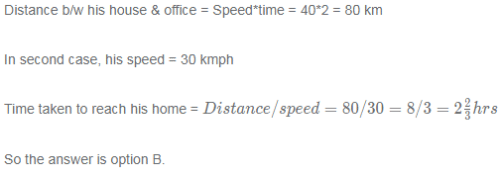RRB Group D Mock Test - 29 - Question 62

A and B invested some money in a business. A invested Rs. 12000 for 8 months while B invested Rs. 8000 for the entire year. Find the ratio of A's share to B's share in the profit?

Detailed Solution for RRB Group D Mock Test - 29 - Question 62

Ratio of shares = (12000*8) : (8000*12) = 96 : 96 = 1 : 1(12000∗8):(8000∗12)=96:96=1:1

So the answer is option C.

RRB Group D Mock Test - 29 - Question 63

Nithya wants to purchase 12 chocolates, 15 pencils, 13 pens. Cost of 1 pencil is 3/-, cost of 2 pens is 10/- and cost of chocolate is 2/-. Then find the total cost ?

Detailed Solution for RRB Group D Mock Test - 29 - Question 63

Cost of 1 pencil is 3/-, cost of 2 pens is 10/- and cost of chocolate is 2/-

Cost of 1 pencil is 3/-, cost of 1 pens is 5/- and cost of chocolate is 2/-

Cost of 12 chocolates, 15 pencils, 13 pens = 12(2)+15(3)+13(5) = 24+45+65 = 134/-

So the answer is option B.

RRB Group D Mock Test - 29 - Question 64

Find LCM of two numbers, whose product is 864 and HCF is 12 ?

Detailed Solution for RRB Group D Mock Test - 29 - Question 64

LCM*HCF = Product of numbers

LCM*12 = 864

LCM = 72

So the answer is option A.

RRB Group D Mock Test - 29 - Question 65

Find the greatest fraction amongst 13/12, 14/13, 15/14 & 16/15 ?

Detailed Solution for RRB Group D Mock Test - 29 - Question 65

13/12= 1.083

14/13= 1.076

15/14= 1.071

16/15=1.066

So 13/12 is greater than all three remaining fractions.

So the answer is option D.

SHORT CUT:

a/b > c/d, if and only if ad > bc.

Lets compare 13/12 & 14/13==> 13*13 = 169 & 14*12 = 168.==> 169>168 So 13/12 > 14/13

Now, compare 13/12 & 15/14 and 13/12 & 16/15 in the same way, we will get 13/12 is the greatest among all four.

So the answer is option D.

RRB Group D Mock Test - 29 - Question 66

123.45 + 6789.01 - 2345.67 = ?

Detailed Solution for RRB Group D Mock Test - 29 - Question 66

123.45 + 6789.01 - 2345.67 = 6912.46 - 2345.67 = 4566.79

So the answer is option C.

RRB Group D Mock Test - 29 - Question 67

Convert 101010101 into decimal system ?

Detailed Solution for RRB Group D Mock Test - 29 - Question 67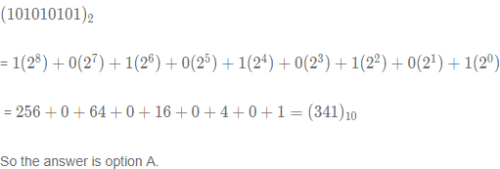RRB Group D Mock Test - 29 - Question 68

In a cricket match, Dhoni scored 46 runs while Raina scored 23 runs. By what percentage did Dhoni outscore Raina?

Detailed Solution for RRB Group D Mock Test - 29 - Question 68

Dhoni's score = 46

Raina's score = 23

Difference = 46 - 23 = 23

answer = \frac{46-23}{23}\times 100 = \frac{23}{23}\times 100 = 1002346−23​×100=2323​×100=100%

So the answer is option C.

RRB Group D Mock Test - 29 - Question 69

An item was purchased for 2000/-. It's price was marked up by 10% and a discount of 20% was given on it. What is the overall loss/profit percentage?

Detailed Solution for RRB Group D Mock Test - 29 - Question 69

CP = 2000/-

SP = (1-0.2)(1+0.1)(2000) = (0.8)(1.1)(2000) = 1760/-

Loss = 2000-1760 = 240

Loss % = \frac{240}{2000}\times 100 = 122000240​×100=12%

So the answer is option D.

RRB Group D Mock Test - 29 - Question 70

Ramesh deposits Rs. 30000 in a bank for three years at simple interest. If the interest earned by him was Rs. 12600, what is the rate of interest?

Detailed Solution for RRB Group D Mock Test - 29 - Question 70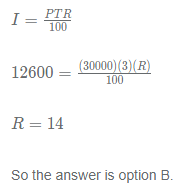RRB Group D Mock Test - 29 - Question 71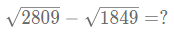Detailed Solution for RRB Group D Mock Test - 29 - Question 71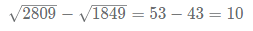So the answer is option A.

RRB Group D Mock Test - 29 - Question 72

Find the length of a platform crossed by a train of length 600meter in 2 minutes, which is travelling with 54kmph?

Detailed Solution for RRB Group D Mock Test - 29 - Question 72

Speed = 54kmph = 54*(5/18) = 15 m/s

Let x be the length of the platform, then

Distance = Speed*time

(x+600) = 15*120

x+600 = 1800

x = 1200

So the answer is option D.

RRB Group D Mock Test - 29 - Question 73

6 years earlier, the ages of Suresh & Ramesh are in the ratio 5:6. Suresh will be 45 years old nine years from now. What is the age of Ramesh 15 years from now?

Detailed Solution for RRB Group D Mock Test - 29 - Question 73

Age of Suresh after 9 years from now is 45 ==> present age of suresh = 45-9 = 36

Let x be the present age of Ramesh

Before 6 years, Age of suresh = 36-6 = 30 & age of ramesh = x-6

Their ratio = 5::6

30/(x-6) = 5/6

x = 42

Age of Ramesh after 15 years = 42+15 = 57

So the answer is option D.

RRB Group D Mock Test - 29 - Question 74

Find the average number of days per month in the year 1994 ?

Detailed Solution for RRB Group D Mock Test - 29 - Question 74

1994 has 365 days

average days per month = \frac{365}{12} = 30.416612365​=30.4166

So the answer is option B.

RRB Group D Mock Test - 29 - Question 75

Find the area(in sq cm) of a regular hexagon of side 4 cm ?

Detailed Solution for RRB Group D Mock Test - 29 - Question 75

Area of regular hexagon =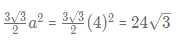So the answer is option C.

RRB Group D Mock Test - 29 - Question 76

Find the distance between A(4, 9) & B(7, 5) ?

Detailed Solution for RRB Group D Mock Test - 29 - Question 76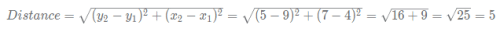So the answer is option D.

RRB Group D Mock Test - 29 - Question 77

Find the no.of cubes of side 2cm, that can be extracted from a cuboid of length 12 cm, breadth 8 cm and height 10 cm ?

Detailed Solution for RRB Group D Mock Test - 29 - Question 77

Volume of cuboid = lbh = 12*8*10 = 960 cm³

Volume of cube = 2³ = 8 cm³

No.of cubes = \frac{960}{8} = 1208960​=120

So the answer is option B.

RRB Group D Mock Test - 29 - Question 78

sin−1x+cos−1x=?(−1≤ x ≤1)

Detailed Solution for RRB Group D Mock Test - 29 - Question 78

For any value of x, sin^{-1}x + cos^{-1}x = \pi/2sin−1x+cos−1x=π/2

Lets take x = 1/2

sin^{-1}(1/2) + cos^{-1}(1/2) = 30 + 60 = 90sin−1(1/2)+cos−1(1/2)=30+60=90°= \pi/2π/2

So the answer is option A.

RRB Group D Mock Test - 29 - Question 79

Find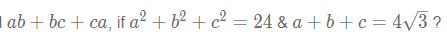Detailed Solution for RRB Group D Mock Test - 29 - Question 79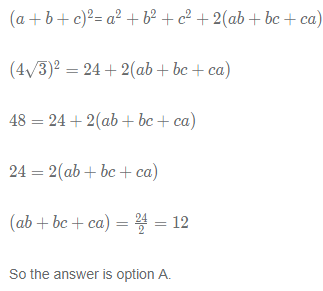RRB Group D Mock Test - 29 - Question 80

A shopkeeper mixed Type-A rice with Type-B rice in the ratio 4:1. Cost prices of Type-A rice and Type-B rice are 40/-per kg and 35/-per kg respectively. By selling the mixture he earned 12% profit. Find the approximate selling price per kg of mixture ?

Detailed Solution for RRB Group D Mock Test - 29 - Question 80

Let the shopkeeper mixed 4 kgs of Type A & 1 kg of Type B.

CP of mixture = 4(40)+1(350 = 195/- per 5 kgs = 195/5 per kg = 39/- per kg

Selling price = 112% of (39) = 1.12(39) = 43.68 \sim∼ 43.5/- per kg

So the answer is option C.

RRB Group D Mock Test - 29 - Question 81

Select the option that is different from the other 3 options

Detailed Solution for RRB Group D Mock Test - 29 - Question 81

ohannesburg is not one of the capitals of South Africa.
Cape town is the legislative capital of South Africa.
Pretoria is the administrative capital of South Africa.
Bloemfontein is the judicial capital of South Africa.

Therefore, option B is the right answer.

RRB Group D Mock Test - 29 - Question 82

Select the option that is different from the other 3 options:

Detailed Solution for RRB Group D Mock Test - 29 - Question 82

Toad is an amphibious animal. Snake, crocodile and lizard are reptiles. Therefore, option B is the right answer.

RRB Group D Mock Test - 29 - Question 83

Which of the following numbers must continue the series?
3, 8, 15, 24, 35, 48, ?

Detailed Solution for RRB Group D Mock Test - 29 - Question 83

1*3 = 3
2*4 = 8
3*5 = 15
4*6 = 24
5*7 = 35
6*8 = 48
7*9 = 63

Therefore, option B is the right answer.

RRB Group D Mock Test - 29 - Question 84

6 friends A, B, C, D, E and F are staying in a 6 storied apartment. The floors are numbered from 1 to 6 - 1 being the lowest and 6 being the highest. A and E live on odd-numbered floors. Neither of them lives on floor 1. D and B live on floors above A. F lives on an even numbered floor. D does not live on the floor between A and E. How many persons live between C and B?

Detailed Solution for RRB Group D Mock Test - 29 - Question 84

A and E live on odd numbered floor but neither of them lives on floor 1. Therefore, A and E must be living on floors 3 and 5, in no particular order.

D and B live on floors above A. Therefore, D and B must be living on floors 4 and 6 in no particular order.

D does not live on the floor between A and E. Therefore, D must be living on floor 6 and B must be living on floor 4. F lives on an even numbered floor. Therefore, F must be living on floor 2 and C must be living on floor 1.

The final arrangement is as follows:

1 - C
2 - F
3 - A/E
4 - B
5 - A/E
6 - D

2 persons live between B and C. Therefore, option B is the right answer.

RRB Group D Mock Test - 29 - Question 85

Which of the following options can be a part of the series given below?
CDG, BFH, FHN, OCR , ?

Detailed Solution for RRB Group D Mock Test - 29 - Question 85

Let us replace the alphabets with numbers.

CDG - (3,4,7)
BFH - (2,6,8)
FHN - (6,8,14)
OCR - (15,3,18)

As we can see, the position of the third letter in the alphabetical series is the sum of the positions of the first 2 letters.
Now, let us evaluate the options.
SDV - (19, 4, 22)
IHQ - (9, 8, 17)
SEY - (19,5,25)
PDU - (16,4,21).

Only IHQ satisfies the condition of the position of the third letter being the sum of the positions of the first 2 letters. Therefore, option B is the right answer.

RRB Group D Mock Test - 29 - Question 86

If SLAIN is coded as TNDMS, what will be TREAD coded as?

Detailed Solution for RRB Group D Mock Test - 29 - Question 86

S + 1 = T
L + 2 = N
A + 3 = D
I + 4 = M
N + 5 = S

Using the same logic,
T + 1 = U
R + 2 = T
E + 3 = H
A+ 4 = E
D + 5 = I

TREAD will be coded as UTHEI. Therefore, option B is the right answer.

RRB Group D Mock Test - 29 - Question 87

If, ‘+’ is coded as ‘&’, ‘*’ is coded as ‘$’, ‘/’ is coded as ‘@’, ‘-‘ is coded as ‘!’then what is the value of 47 ! 252 @ 7 & 16$ 3?

Detailed Solution for RRB Group D Mock Test - 29 - Question 87

47 ! 252 @ 7 & 16 \$ 3 would mean 47 - (252/7) + 16 * 3 = 47 - 36 + 48 = 59
Hence, option B is right choice.

RRB Group D Mock Test - 29 - Question 88

If 253 & 94 = 159 and 26 # 11 = 286, then what is the value of 326 & 19 # 8?

Detailed Solution for RRB Group D Mock Test - 29 - Question 88

326 & 19 # 8 = 326 - (19*8) = 174
Hence, option C is the right answer.

RRB Group D Mock Test - 29 - Question 89

Which of the following Venn diagrams represents the relationship between Moon, Satellite, Planet, Pluto, Mars and Earth?

Detailed Solution for RRB Group D Mock Test - 29 - Question 89

Mars and Earth are planets. Moon is a satellite. Pluto is neither a planet nor a satellite.
The diagram must contain 2 non-intersecting circles inside a large circle (Planets), 1 small circle inside a large circle (Moon is a satellite) and 1 isolated circle (to represent Pluto). Only option C satisfies these conditions and hence, it is the right answer.

RRB Group D Mock Test - 29 - Question 90

If square represents singers, circle represents swimmers and hexagon represents dancers, the number of swimmers who are dancers but not singers is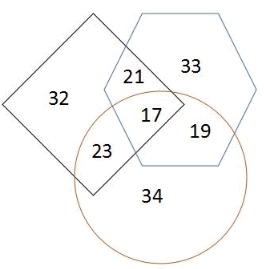Detailed Solution for RRB Group D Mock Test - 29 - Question 90

Number of swimmers who are dancers but not singers is represented by the area of the circle common to the hexagon but not common to the square. As we can see from the diagram, 19 people are swimmers and dancers but not singers. Therefore, option A is the right answer.

RRB Group D Mock Test - 29 - Question 91

In the question below a statement is followed by two assumptions numbered I and II. An assumption is something supposed or taken in for granted. You have to consider the statement and the following assumptions and then decide which of the assumptions is implicit in the statement.

Statement:

A riot broke out in a college over an issue. Students went on a rampage and destroyed properties. The college management immediately declared a week holiday to control the problem.

Assumptions:

I. The students perpetrated the entire events to get some holidays.
II. Providing some time will help to cool down the temper of the students.

Detailed Solution for RRB Group D Mock Test - 29 - Question 91

The purpose of declaring a holiday is to avoid further damage. Declaring holidays will prevent students from gathering at a common spot, which is conducive to a violent riot to perpetrate. Therefore, the purpose of declaring a holiday is to get some time to delve into the issue with a sane mind. Hence, only assumption II is implicit and hence, option B is the right answer.

RRB Group D Mock Test - 29 - Question 92

In the question below a statement is followed by two assumptions numbered I and II. An assumption is something supposed or taken in for granted. You have to consider the statement and the following assumptions and then decide which of the assumptions is implicit in the statement.

Statement:

The Government of a nation has recently announced that currency notes of large denominations will be invalid in an effort to curtail black money. Also, it has introduced a maximum cap on the amount of gold that one can store in one’s house.

Assumptions:

I: Currencies of large denominations make the process of storing black money easier.
II: Currencies are the only form in which black money is stashed illegally by people.

Detailed Solution for RRB Group D Mock Test - 29 - Question 92

The Government declared currencies of large denominations invalid under the assumption that it makes the process of storing black money easier. Therefore, assumption I is implicit.

The fact that the government has put a cap on the amount of gold that can be stored implies that the government recognizes the fact that black money can be stored in forms other than currencies too. Therefore, assumption II is not implicit. Only assumption I is implicit and hence, option A is the right answer.

RRB Group D Mock Test - 29 - Question 93

Select the option that bears the same relationship as the terms given in the question:
AN : CP :: ER : ?

Detailed Solution for RRB Group D Mock Test - 29 - Question 93

A and N have 12 letters between them. The next term starts with C ( A letter that is 2 places after A in the alphabetical series).

C and P have 12 letters between them in the alphabetical series.

E and R have 12 letters between them in the alphabetical series. The next term must start with G.
G and T have 12 letters between them. Therefore, GT must be the next term. Hence, option B is the right answer.

RRB Group D Mock Test - 29 - Question 94

Select the option that bears the same relationship as the words given in the question:Bangladesh: Dhaka :: Myanmar : ?

Detailed Solution for RRB Group D Mock Test - 29 - Question 94

Dhaka is the capital of Bangladesh. Similarly, Naypyidaw is the capital of Myanmar. The capital of Myanmar was changed from Rangoon to Naypyidaw in 2005. Therefore, option D is the right answer.

RRB Group D Mock Test - 29 - Question 95

Select which of the statements given will be sufficient to solve the problem given below:

5 friends A, B, C, D, and E have 2, 3, 5,7 and 9 coins with them, not necessarily in the same order. The difference between the number of coins with B and C is equal to the number of coins with D. The sum of the number of coins with B and C is equal to the number of coins with E. Which of the following statements will be sufficient to determine the number of coins with each of the 5 persons uniquely?

Statement I: The sum of the number of coins with D and E is 1 greater than the number of coins with A.
Statement II: B has more coins than D

Detailed Solution for RRB Group D Mock Test - 29 - Question 95

Let the number of coins with A, B, C, D and E be a, b, c, d and e respectively.
b - c = d
b and c can be (2,5), (3,5) , (5,7) and (7,9) in any order.
b + c = e
b and c can only be (2,5).

Therefore, the number of coins with D must be 3, E must be 7 and A must be 9.
The number of coins with B and C can be (2,5) in any order.

Statement I states that the sum of the number of coins with D and E is 1 greater than the number of coins with A. This condition does not help us to fix the number of coins with B and C. Therefore, statement I is insufficient.

Statement II states that B has more coins than D. Therefore, B must have 5 coins and C must have 2 coins. Therefore, statement II alone is sufficient to determine the number of coins with each of the 5 persons uniquely. Therefore, option B is the right answer.

RRB Group D Mock Test - 29 - Question 96

If ‘Flights are avians’ is coded as ‘pa ru da’ and ‘Birds are not avians’ is coded as ‘ru pa la na’ which of the following cannot be the code for ‘Birds are flights’?

Detailed Solution for RRB Group D Mock Test - 29 - Question 96

As we can see, the code for ‘flights’ is ‘da’. The code for ‘are’ and ‘avians’ is ‘pa’ and ‘ru’ in any order. Similarly, the code for ‘Birds’ and ‘not’ is ‘na’ and ‘la’ in any order. Therefore, the code for ‘Birds are flights’ must contain ‘da’, exactly one among ‘pa’ and ‘ru’ and exactly one among ‘na’ and ‘la’. Option A violates this condition and hence, option A is the right answer.

RRB Group D Mock Test - 29 - Question 97

If ‘Camera is on’ is coded as ‘ pa ba hu’ and ‘DSLR is a camera’ is coded as ‘od ba di pa’, which of the following cannot be the code for ‘A DSLR is on a Camera’?

Detailed Solution for RRB Group D Mock Test - 29 - Question 97

The code for ‘camera’ and ‘is’ is ‘pa’ and ‘ba’ in any order. The code for ‘DSLR’ and ‘a’ must be ‘od’ and ‘di’ in any order. One of these 2 codes must be used twice and the other must be used once. All other codes must be used exactly once. Option D violates this condition and hence, option D is the right answer.

RRB Group D Mock Test - 29 - Question 98

If ‘How are you’ is coded as ‘tr we me’, ‘You are fine’ is coded as ‘me tr gu’, and ‘I am fine’ is coded as ‘od gu ka’, which of the following can be the code for ‘How fine am I’?

Detailed Solution for RRB Group D Mock Test - 29 - Question 98

From the second and the third sentences, we can infer that the code for ‘fine’ is ‘gu’. The code for ‘I’ and ‘am’ is ‘od’ and ‘ka’ in any order. Similarly, the code for ‘You’ and ‘are’ is ‘me’ and ‘tr’ in any order. The code for ‘how’ is ‘we’.

The code for ’How fine am I’ must consist of ‘we’, ‘gu’ and both ‘od’ and ‘ka’. Only option C satisfies this condition. Hence, option C is the right answer.

RRB Group D Mock Test - 29 - Question 99

Preetha starts from her home and walks 20 m towards the West. She takes a right turn and walks 15 m. Then, she takes a left turn and walks 20 m. Finally, she takes a 45 m. How far is she from the starting point and which direction is she facing?

Detailed Solution for RRB Group D Mock Test - 29 - Question 99

The given conditions can be expressed diagrammatically as follows.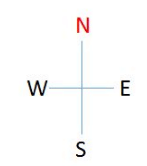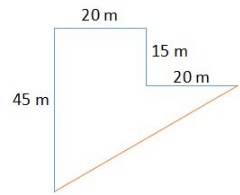Total East-West distance travelled - 20 + 20 = 4020+20=40m
Total North-South distance travelled - 45 - 15 = 3045−15=30m

Using Pythagoras theorem, we can find the distance between the starting point and the ending point.
Distance = \sqrt{ 40^2 + 30^2}402+302​
= \sqrt {2500}2500​
= 5050 m.

Also, Preetha is facing the South. Therefore, option A is the right answer.

RRB Group D Mock Test - 29 - Question 100

There are 5 members in a family - A, B, C, D and E. The family spans 3 generations and contains 2 married couples. E is the son of A and husband of B. E is related to C the same way D is related to B. How is D related to A?

Detailed Solution for RRB Group D Mock Test - 29 - Question 100

E is the son of A and husband of B. Therefore, B must be a female member. C is related to E the same way B is related to D. Therefore, E must be the son of C and D must be the son of B. D is the grandson of A.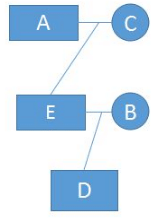Therefore, option B is the right answer.

## RRB (Group D) - Mock Tests & Previous Year Papers

1 docs|48 tests
 Use Code STAYHOME200 and get INR 200 additional OFF Use Coupon Code
Information about RRB Group D Mock Test - 29 Page
In this test you can find the Exam questions for RRB Group D Mock Test - 29 solved & explained in the simplest way possible. Besides giving Questions and answers for RRB Group D Mock Test - 29, EduRev gives you an ample number of Online tests for practice

1 docs|48 tests